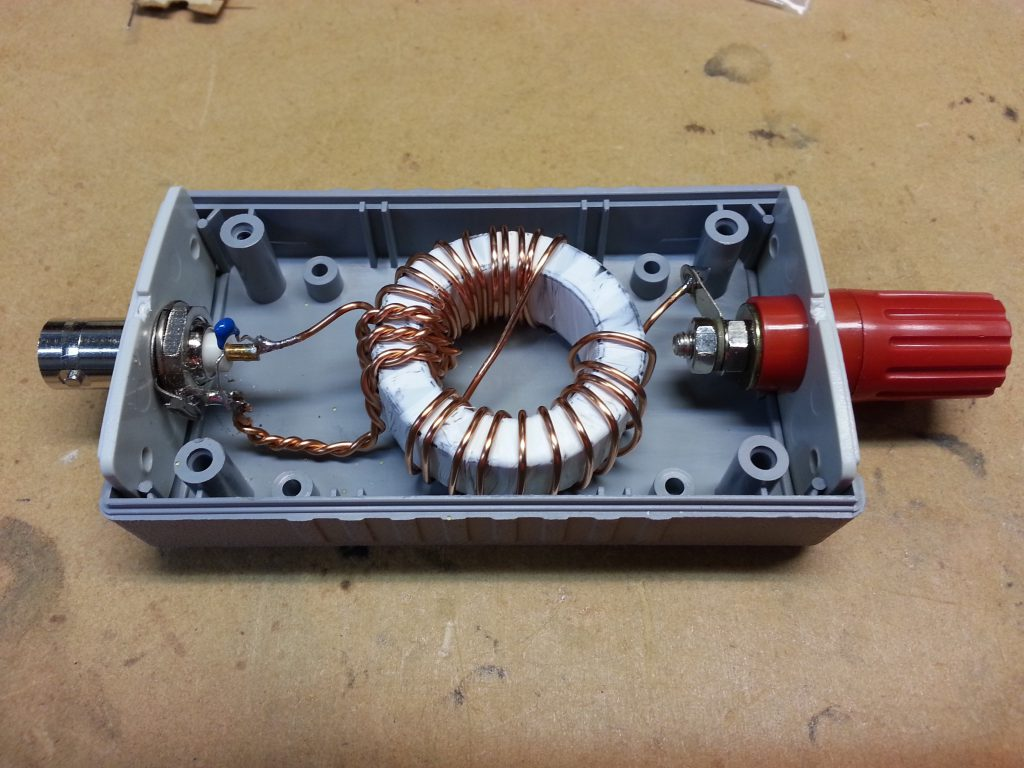# End fed half wave matching – voltage rating of compensation capacitors

The so-called End Fed Half Wave antenna system has become more popular, particularly in the form of a broadband matching transformer in combination with a wire operated harmonically over perhaps three octaves (eg 7, 14, 21, 28MHz).

The broadband transformer commonly uses a medium µ ferrite toroid core, and a turns ratio of around 8:1. Flux leakage results in less than the ideal n^2 impedance transformation, and a capacitor is often connected in parallel with the 50Ω winding to compensate the transformer response on the higher bands.

David, VK3IL posted EFHW matching unit in which he describes a ferrite cored transformer matching unit that is of a common / popular style.Above is David’s pic of his implementation. It is a FT140-43 toroid with 3 and 24t windings and note the 150pF capacitor in shunt with the coax connector.

The article End fed matching – analysis of VK3IL’s measurements gives the following graph showing the effects of compensation for various resistive loads.An online expert opined in the context of a 100W antenna system use a 3Kv or better cap across the input to help with SWR on the higher bands.

Since the advice was offered with no explanation, it is questionable. Whilst a 3kV rated capacitor is likely to be adequate, they are quite hard to obtain and it is worth considering a rational design rather than a figure picked out of the air with no supporting justification.

It would be naive to use a capacitor rated for no more than the nominal working voltage, safety factors are appropriate. Lets declare two safety factors to be applied:

• VSWR up to 3:1 which could result in voltage of 3^0.5 times nominal; and
• an overall 100% increase in the voltage rating for conservative reserve.

The peak working voltage for 100W in 50Ω is given as (2*P*R)^0.5=(2*100*50)^0.5=100V. Applying a safety factor of 2*3^0.5, we get 346V.

So, for adequate voltage breakdown we would select an off the shelf component with a rating of greater then 346V, a common value for these caps is 600V.

Clearly the online expert’s recommendation of 3kV is over the top, 500% of what is prudent, a further reserve of 14dB which isn’t necessarily a bad thing… except that a 3kV low loss component is much harder to find.

For lower power, lower voltage withstand is adequate, eg on the same basis, 77V or better is sufficient for a nominal 5W.

I mentioned loss!

Lots of published articles show the use of inexpensive ‘moulded mud’ ceramic capacitors in this application at low power levels.

Not only have these low grade capacitors low voltage ratings (commonly 50V), but the dielectric is lossy, capacitor Q might not be better than 50.

Lets consider the loss in a 150pF shunt capacitor with Q=50 at 30MHz with 100W input to the matching network. Xc=1/(2*π*f*c)=35Ω, and by virtue of Q=50, it has an equivalent shunt loss resistance of Q*Xc=50*35=1750Ω. The voltage impressed on that capacitor at 100W in 50 is (P*R)^0.5=(100*50)^0.5=70Vrms. The power dissipated in the capacitor is E^2/R=70^2/1750=2.8W… way in excess of what is safe for a tiny capacitor.

## Conclusions

Voltage rating of the compensation capacitor is easy to calculate, and worth the effort as low loss capacitors suited to the application may be hard to find, especially with higher voltage rating.

These capacitors should be of a type that has low loss at the frequencies in use.